# Division - 5th grade (10y) - math problems

#### Number of problems found: 109

• DivisionWhich number in division 16 give 12 and the rest 3?
• Quotient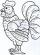Determine the quotient (q) and the remainder (r) from division numbers 100 and 8. Take the test of correctness.
• MilimetersHow many millimeters is 1/4 meters?
• The resultHow many times I decrease the number 1632 to get the result 24?
• Parenthesis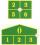Fill in the examples correctly brackets: A) 7.9+12:3-2 =23 B) 7.9+12:3-2=75How much and how many times is 72.1 greater than 0.00721?
• Expression plus minusEvaluate expression: (-1)2 . 12 – 6 : 3 + (-3) . (-2) + 22 – (-3) . 2
• Bucket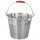How many 5-liter buckets do you have to pour into a 0.2 m3 container to make it full?
• Cakes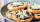On the table was 56 cakes. When Peter went home, leaving them eight times less. How many cakes left?
• Holidaymakers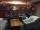A total of 94270 holidaymakers spent part of their holiday in 50 hotels a year. On average, how many vacationers are there for one hotel?
• Value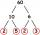Determine the value of the following exspressions: a) (23-25)·(4-5) b) (97-123):(18+8)
• Wallpaper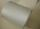Salesman account for 2.3 meters of wallpaper 149.50 Kc. How much cost 1 meter of wallpaper?
• Minutes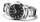Write as fraction in basic form which part of the week is 980 minutes.
• Products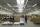15 products are 48 € more expensive than 12 products. How many euros will it cost five same products?
• Soda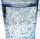A pack of 12 sodas cost 5.04. What is the unit rate in dollars per soda?
• Trio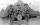56 children lined up in groups of three. How many children did not create a trio?
• Cake 71/3 of a cake shared with 4 people. What share of the whole cake has each people?
• Women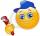In an company of 1050 employees are 2/3 women. How many women work in the company?
• Math classificationIn 3A class are 27 students. One-third got a B in math and the rest got A. How many students received a B in math?
• A koala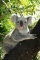A koala lives to be 14 years old in the wild. How much longer will a 2 year old koala probably live?

Do you have an interesting mathematical word problem that you can't solve it? Submit a math problem, and we can try to solve it.

We will send a solution to your e-mail address. Solved examples are also published here. Please enter the e-mail correctly and check whether you don't have a full mailbox.

Please do not submit problems from current active competitions such as Mathematical Olympiad, correspondence seminars etc...